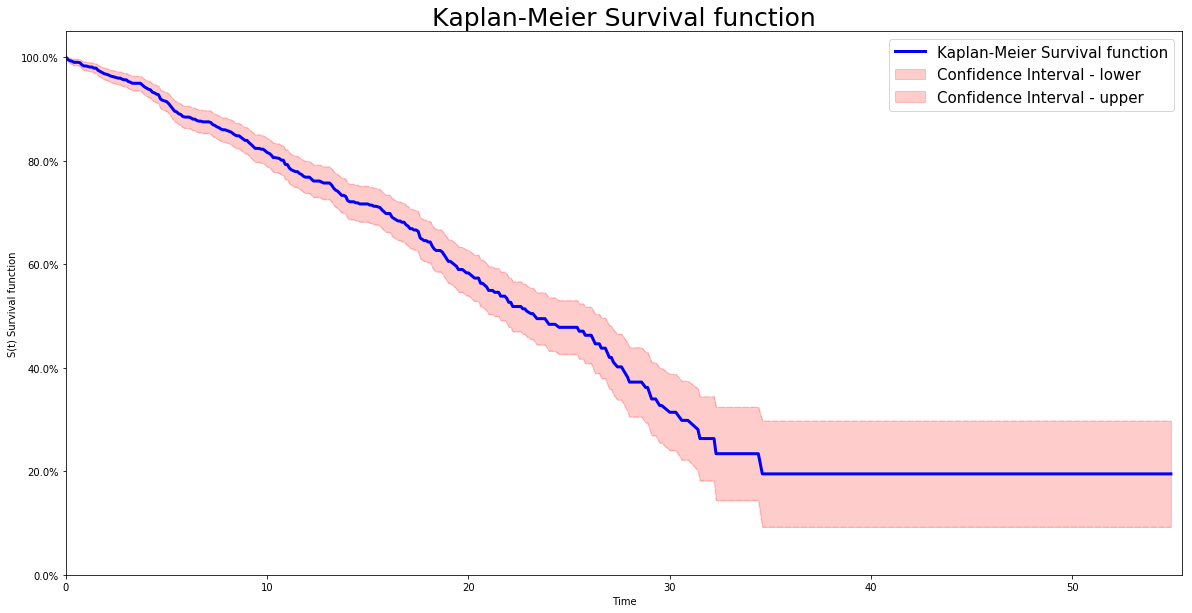# Kaplan Meier model

The Kaplan–Meier estimator, introduced by Kaplan et al. in 1958, also known as the product limit estimator, is a non-parametric model used to estimate the survival function of a cohort.

## Instance

To create an instance, use pysurvival.models.non_parametric.KaplanMeierModel.

## Attributes

• cumulative_hazard: array-like -- representation of the cumulative hazard function of the model
• hazard: array-like -- representation of the hazard function of the model
• survival: array-like -- representation of the Survival function of the model
• times: array-like -- representation of the time axis of the model
• time_buckets: array-like -- representation of the time axis of the model using time bins, which are represented by $[ t_{k-1}, t_k )$

## Methods

fit - Fit the estimator based on the given parameters

fit(T,  E, weights = None, alpha=0.05)


Parameters:

• T : array-like -- target values describing the time when the event of interest or censoring occurred.

• E : array-like -- values that indicate if the event of interest occurred i.e.: E[i]=1 corresponds to an event, and E[i] = 0 means censoring, for all i.

• weights : array-like (default = None) -- array of weights that are assigned to individual samples. If not provided, then each sample is given a unit weight.

• alpha: float (default = 0.05) -- confidence level

Returns:

• self : object

predict_density - Predicts the probability density function $p(t)$ at a specified time t

predict_density(t)


Parameters:

• t: double -- time at which the prediction should be performed.

Returns:

• density: double -- prediction of the probability density function at t

predict_hazard - Predicts the hazard function $h(t)$ at a specified time t

predict_hazard(t)


Parameters:

• t: double -- time at which the prediction should be performed.

Returns:

• hazard: double -- prediction of the hazard function at t

predict_survival - Predicts the survival function $S(t)$ at a specified time t

predict_survival(t)


Parameters:

• t: double -- time at which the prediction should be performed.

Returns:

• survival: double -- prediction of the survival function at t

## Example

# Importing modules
import numpy as np
from matplotlib import pyplot as plt
from pysurvival.models.non_parametric import KaplanMeierModel
from pysurvival.utils.display import display_non_parametric
# %matplotlib inline #Uncomment when using Jupyter

# Generating random times and event indicators
T = np.round(np.abs(np.random.normal(10, 10, 1000)), 1)
E = np.random.binomial(1, 0.3, 1000)

# Initializing the KaplanMeierModel
km_model = KaplanMeierModel()

# Fitting the model
km_model.fit(T, E, alpha=0.95)

# Displaying the survival function and confidence intervals
display_non_parametric(km_model)Figure 1 - Representation of the Kaplan Meier Survival function